# Aqueous Solutions Solutions Solutions are defined as homogeneous

• Slides: 14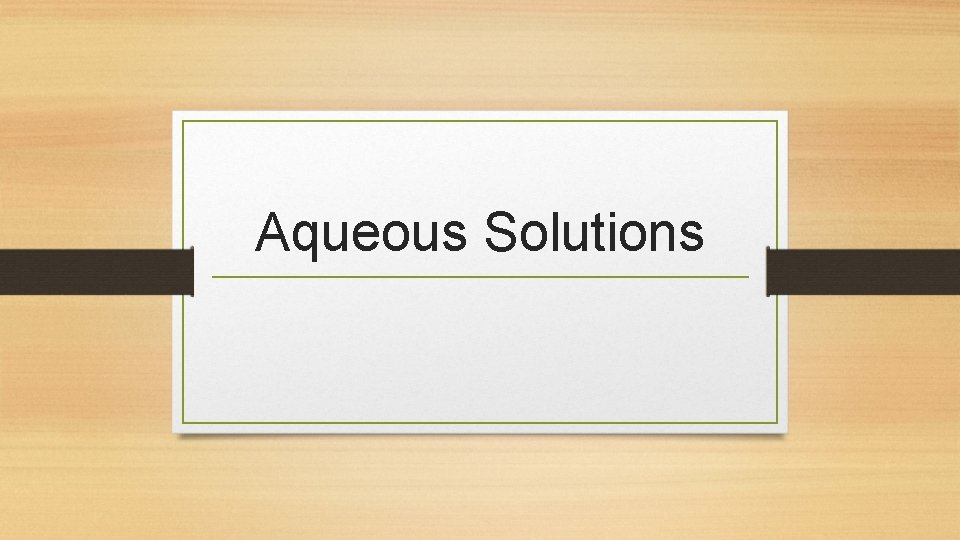Aqueous Solutions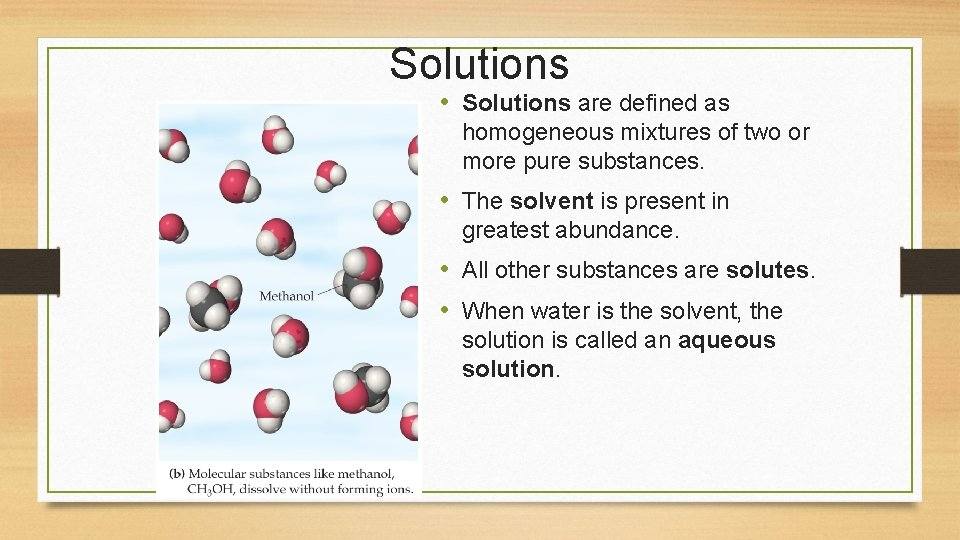Solutions • Solutions are defined as homogeneous mixtures of two or more pure substances. • The solvent is present in greatest abundance. • All other substances are solutes. • When water is the solvent, the solution is called an aqueous solution.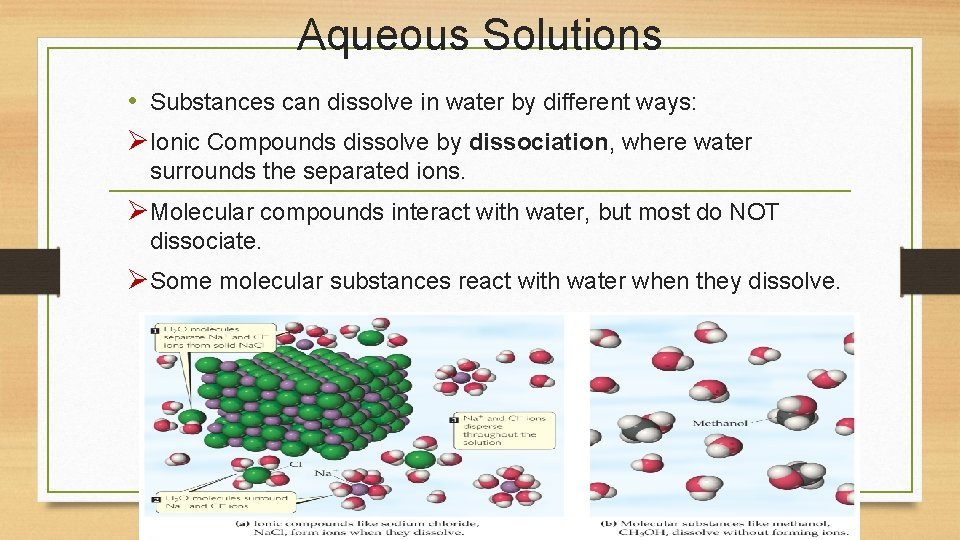Aqueous Solutions • Substances can dissolve in water by different ways: ØIonic Compounds dissolve by dissociation, where water surrounds the separated ions. ØMolecular compounds interact with water, but most do NOT dissociate. ØSome molecular substances react with water when they dissolve.Electrolytes and Nonelectrolytes • An electrolyte is a substance that dissociates into ions when dissolved in water. • A nonelectrolyte may dissolve in water, but it does not dissociate into ions when it does so.Electrolytes • A strong electrolyte dissociates completely when dissolved in water. • A weak electrolyte only dissociates partially when dissolved in water. • A nonelectrolyte does NOT dissociate in water.Solubility of Ionic Compounds • Not all ionic compounds dissolve in water. • A list of solubility rules is used to decide what combination of ions will dissolve.Precipitation Reactions When two solutions containing soluble salts are mixed, sometimes an insoluble salt will be produced. A salt “falls” out of solution, like snow out of the sky. This solid is called a precipitate.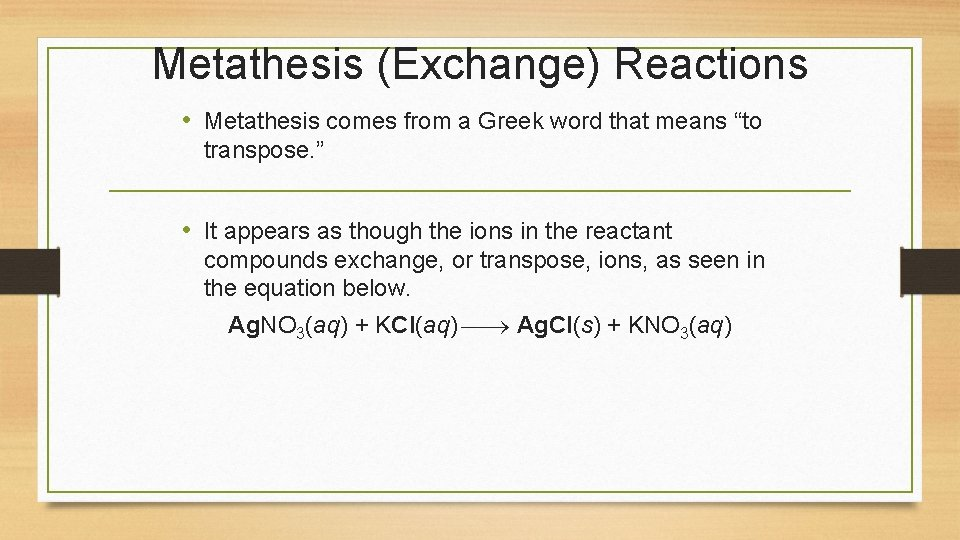Metathesis (Exchange) Reactions • Metathesis comes from a Greek word that means “to transpose. ” • It appears as though the ions in the reactant compounds exchange, or transpose, ions, as seen in the equation below. Ag. NO 3(aq) + KCl(aq) Ag. Cl(s) + KNO 3(aq)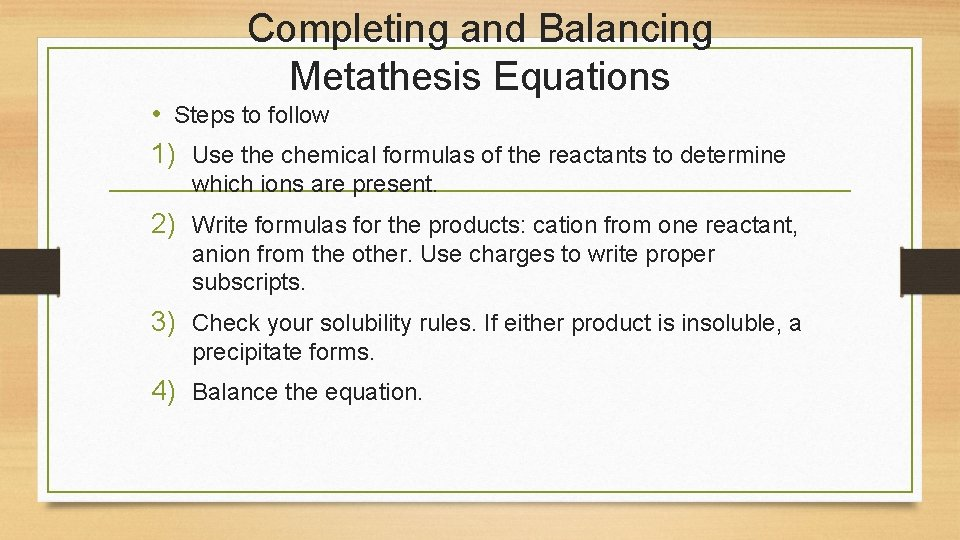Completing and Balancing Metathesis Equations • Steps to follow 1) Use the chemical formulas of the reactants to determine which ions are present. 2) Write formulas for the products: cation from one reactant, anion from the other. Use charges to write proper subscripts. 3) Check your solubility rules. If either product is insoluble, a precipitate forms. 4) Balance the equation.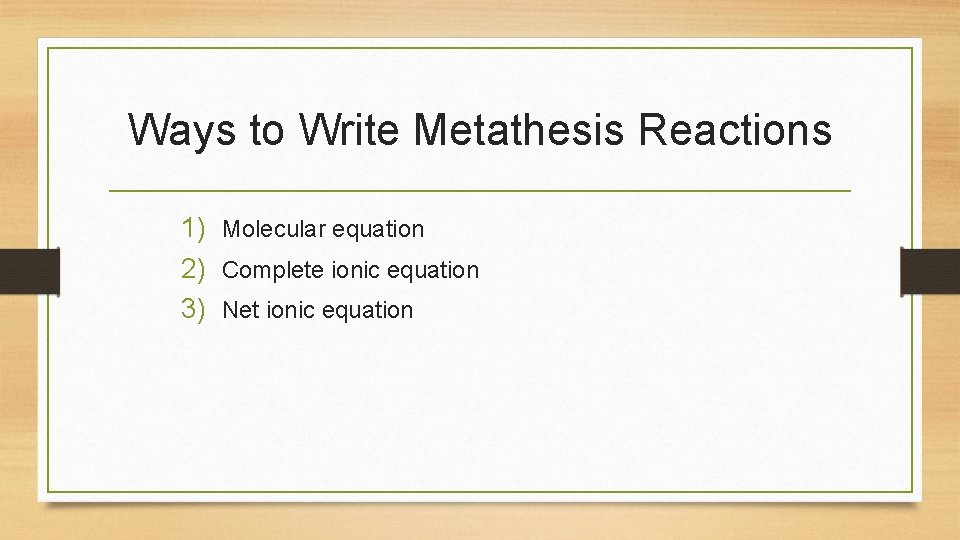Ways to Write Metathesis Reactions 1) Molecular equation 2) Complete ionic equation 3) Net ionic equation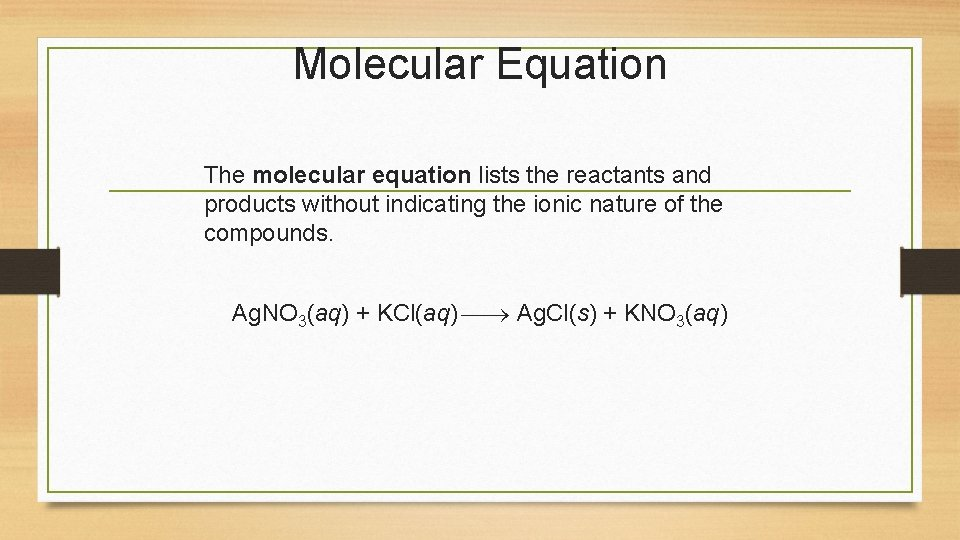Molecular Equation The molecular equation lists the reactants and products without indicating the ionic nature of the compounds. Ag. NO 3(aq) + KCl(aq) Ag. Cl(s) + KNO 3(aq)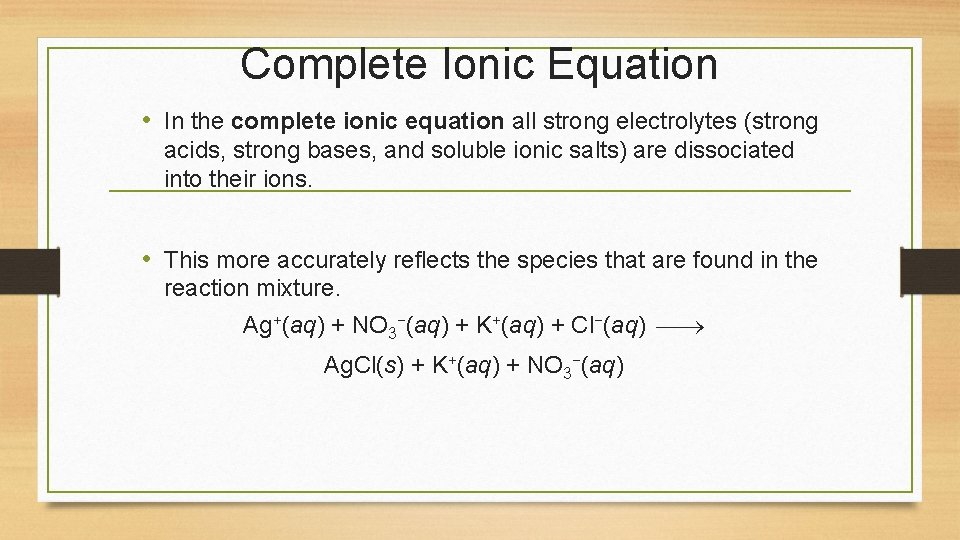Complete Ionic Equation • In the complete ionic equation all strong electrolytes (strong acids, strong bases, and soluble ionic salts) are dissociated into their ions. • This more accurately reflects the species that are found in the reaction mixture. Ag+(aq) + NO 3−(aq) + K+(aq) + Cl−(aq) Ag. Cl(s) + K+(aq) + NO 3−(aq)Net Ionic Equation • To form the net ionic equation, cross out anything that does not change from the left side of the equation to the right. • The ions crossed out are called spectator ions, K+ and NO 3−, in this example. • The remaining ions are the reactants that form the product— an insoluble salt in a precipitation reaction, as in this example. Ag+(aq) + NO 3−(aq) + K+(aq) + Cl−(aq) Ag. Cl(s) + K+(aq) + NO −(aq) 3Writing Net Ionic Equations 1. Write a balanced molecular equation. 2. Dissociate all strong electrolytes. 3. Cross out anything that remains unchanged from the left side to the right side of the equation. 4. Write the net ionic equation with the species that remain.